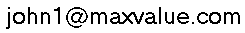"A good science and engineering decision is a good business decision."

Decision Precision training and consulting

The following link is to an Acrobat (pdf) file with 10 questions about decision analysis (DA) and 10 questions about modeling in Excel.  Persons who have recently attended Petroleum Risks and Decision Analysis (PRD) should be able to answer most DA questions easily.

Or, if you prefer, you may sent your numbered answers to us in an email.

If you are unsure about your answers or simply wish to check them, e-mail your answers and you will receive back an answer sheet. A bonus for submitting your answers (useful data for us). We will also send you another 11 DA practice questions (with answers). Use a friend's e-mail account if you wish to remain anonymous.

Send your answers to:Multiple Choice. Select the letter that best answers the question.

PART I. DECISION ANALYSIS

1. For a positively-skewed distribution, what is the proper sequence?
a) mean < median < mode
b) mode < P50 < median
c) mode < median < mean
d) mean < mode < median

2. If a corporation’s goal is to maximize value, the decision policy that best achieves this is choosing the alternative having the highest:
a) most likely value
b) P50 value (median)
c) NPV
d) expected value NPV

3. Decision trees are generally better than Monte Carlo simulation for which type of problem?
a) value of information problems
b) portfolio problems
c) probabilistic cash flow analysis
d) modeling material flows

4. The risk tolerance coefficient used in an exponential utility function for risk policy represents:
a) a scaling factor for risk aversion
b) the maximum amount the company would risk on any one project
c) the company’s net worth
d) the maximum amount the company is willing to pay to avoid risk

5. Latin hypercube sampling applies to:
a) collecting data samples, as in field studies
b) reducing the time to convergence in Monte Carlo simulation
c) method of correlating dependent chance events
d) method of correlating independent chance events

6. The chronology or sequence of nodes in a decision tree generally should follow:
a) Decision. nodes first, then chance events
b) the historical sequence of nature
c) the sequence of the project
d) independent chance events first

7. If P(AB) &qe; 0 then
a) A and B are independent
b) A and B are not mutually exclusive
c) A and B are dependent
d) none of the above

8. Which representation shows the importance of input variables contributing to outcome uncertainty?
b) influence diagram
c) scatter diagram of input variables
d) joint probability table

9. Which is used to revise probabilities based upon new, imperfect information?
a) influence diagram
c) stochastic inversion
d) Bayes’ rule

10. Which is the most useful statistic to measure uncertainty?
a) correlation coefficient
b) range
c) standard deviation
d) median

PART II. MICROSOFT® EXCEL®

1. The Excel program uses what discounting assumption in its NPV function?
a) continuous cashflows
b) cashflows at period starts
c) cashflows at period ends
d) cashflows at mid-periods

2. Algebra for the Excel’s Boolean AND function is about the same as:
a) summation of 0s and 1s
b) multiplication of 0s and 1s
c) addition of 0s and 1s
d) ⊕ operator

3. If cell B3 contains a logic function, then an expression equivalent to =IF(B3,10,5)is:
a) = B3*(10)+(1-B3)*5
b) = B3*10-(1-B3)*5
c) = B3*10
d) none of the above

4. Business models often use the EXP function for:
a) adding an explanatory note to a cell
b) calculating x to the nth power
c) calculating a projection that converges exponentially
d) raising e to a power, where e is the natural log base

5. The difference between Excel’s LN and LOG functions is:
a) LN is the inverse of LOG.
b) LN is the complement of LOG.
c) LN and LOG are equivalent.
d) LN and LOG are the same except for the reference base

6. Excel’s Goal Seek capability:
a) matches a calculation outcome to a specified value by changing one of the input cells
b) optimizes (or minimizes) a calculation cell by changing one or more input parameters
c) recognizes the satisficing condition that all goals have been satisfied
d) finds a combination of decision variables that satisfies all goals or constraints for the problem

7. Defining cell and range names is useful mostly to:
a) provide meaningful labels for charts
b) provide meaningful names to report and chart headings
c) reduce formula errors
d) drawing arrows to where-used on a worksheet

8. Excel evaluates which function first?
b) multiplication
c) division
d) exponentiation

9. An Excel IF statement is useful for:
b) conditional branching logic
c) specifying a font color
d) Boolean “OR” operation

10. In Excel IF statements, true may be represented by:
a) True or True()
b) 1
c) -1
d) all of the above

End of Quiz